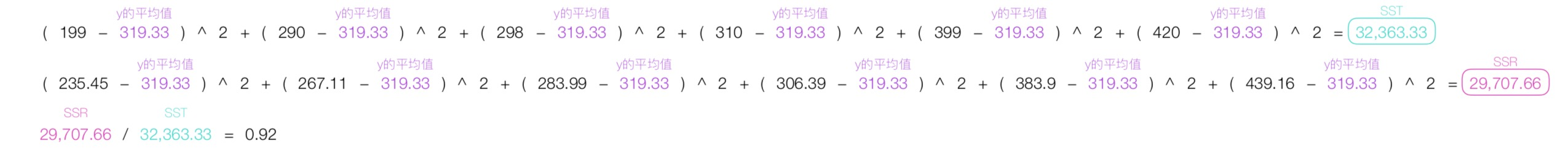机器学习的定义

• 非正式定义：在不直接针对问题进行编程的情况下，赋予计算机学习能力的一个研究领域。
• 正式定义：对于一个计算机程序来讲，给他一个任务T和一个性能测量方法P，如果在经验E的影响下P对T的测量结果得到了改进，那么就说该程序从E中得到了学习。

机器学习四大内容简述

• 监督学习（Supervised Learning）
• 学习理论（Learning Theory）
• 无监督学习（Unsupervised Learning）
• 强化学习（Reinforcement Learning）

监督学习（Supervised Learning）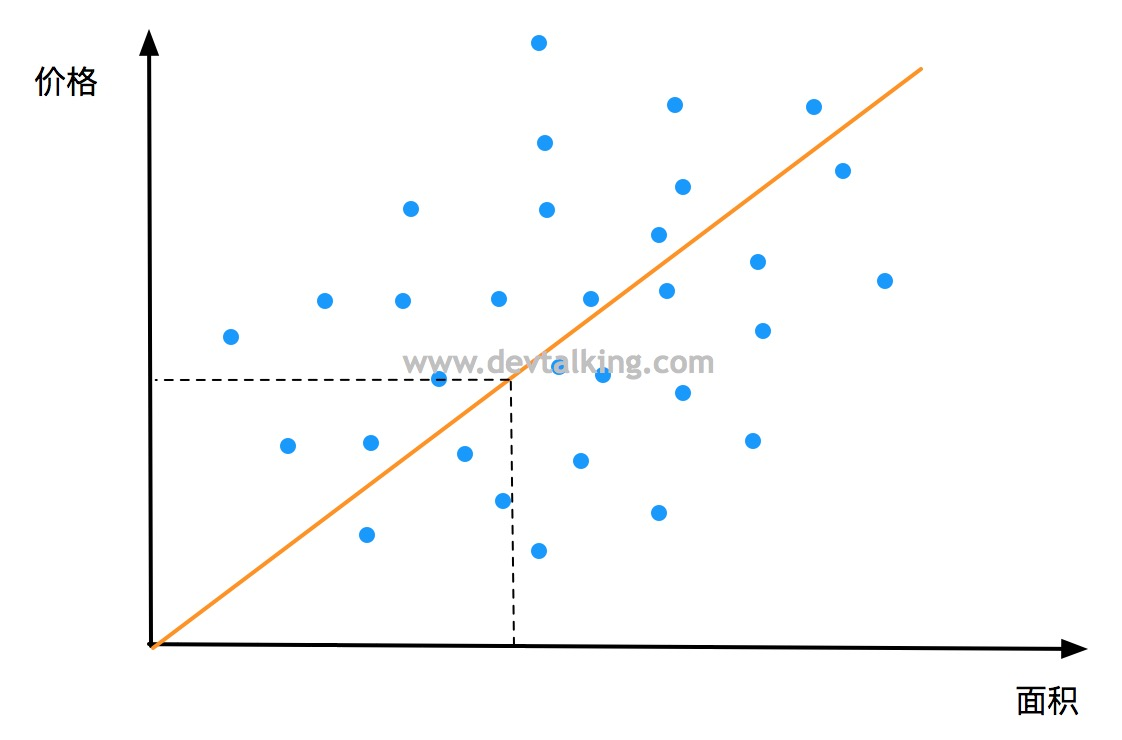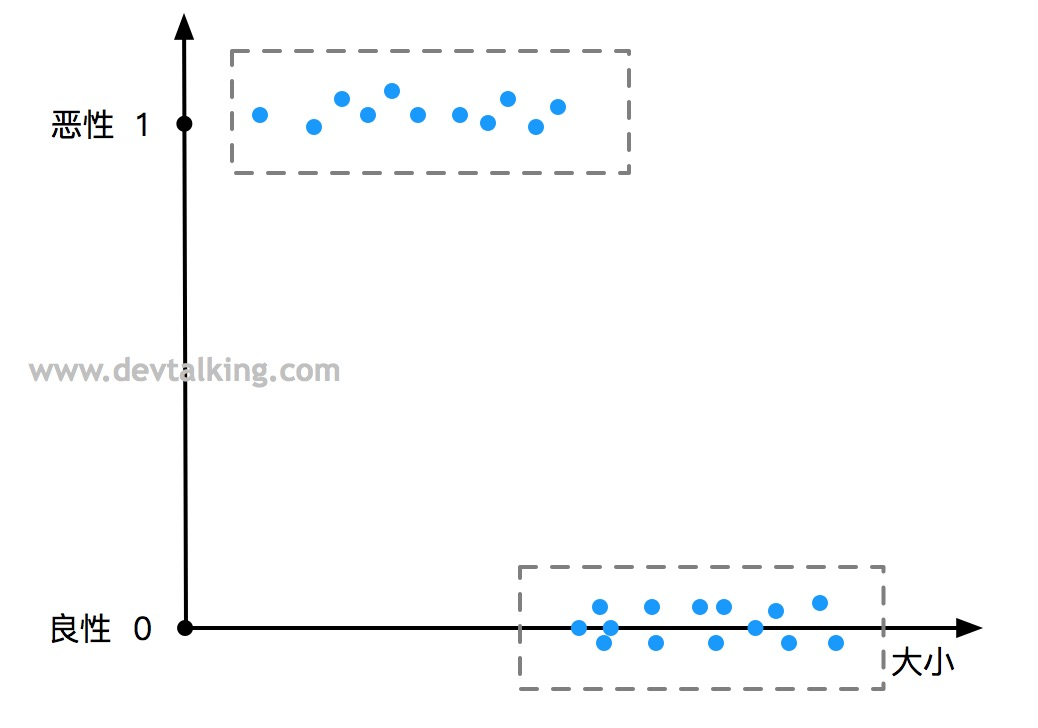监督学习

线性回归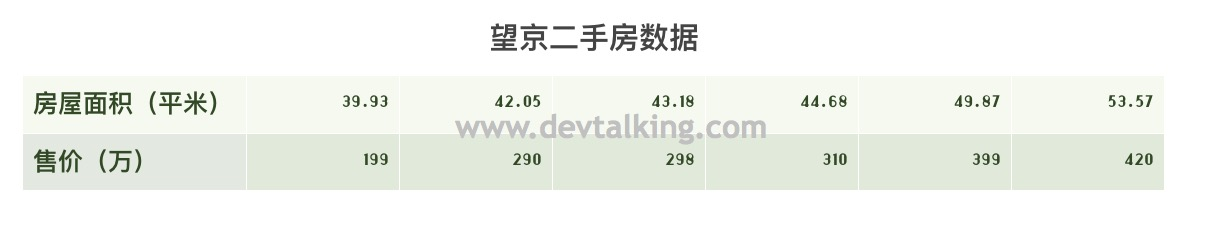如何画出拟合直线

$$(y _ {1实际} - y_{1预测})^2 + (y_{2实际}- y_{2预测})^2 + … + (y_{n实际} - y_{n预测})^2$$

$$F(a,b) = \sum_{i=1}^n(y_i-(ax_i + b))^2$$

如何求函数的最小值

导数与斜率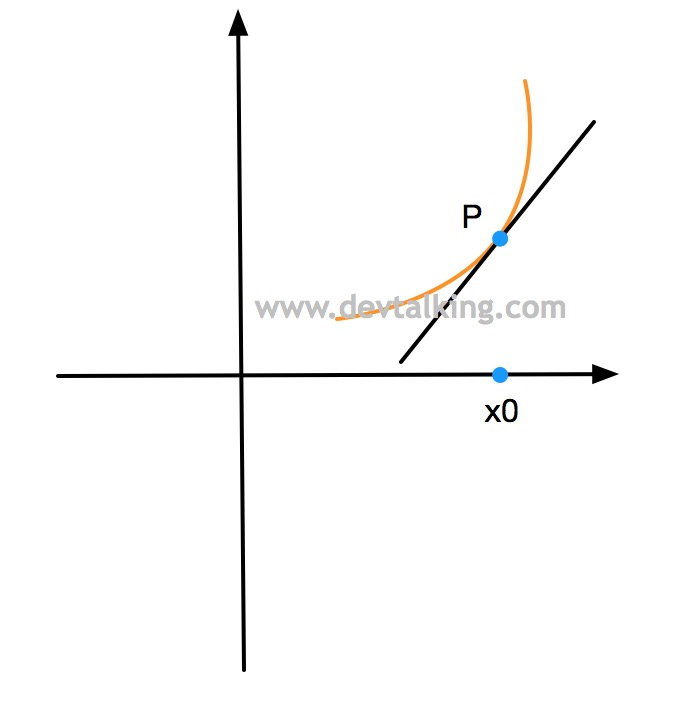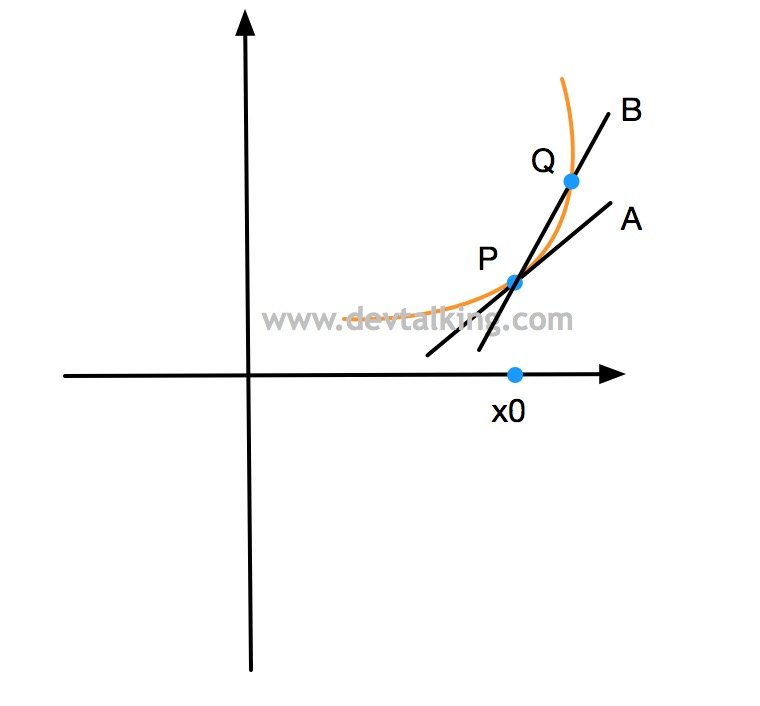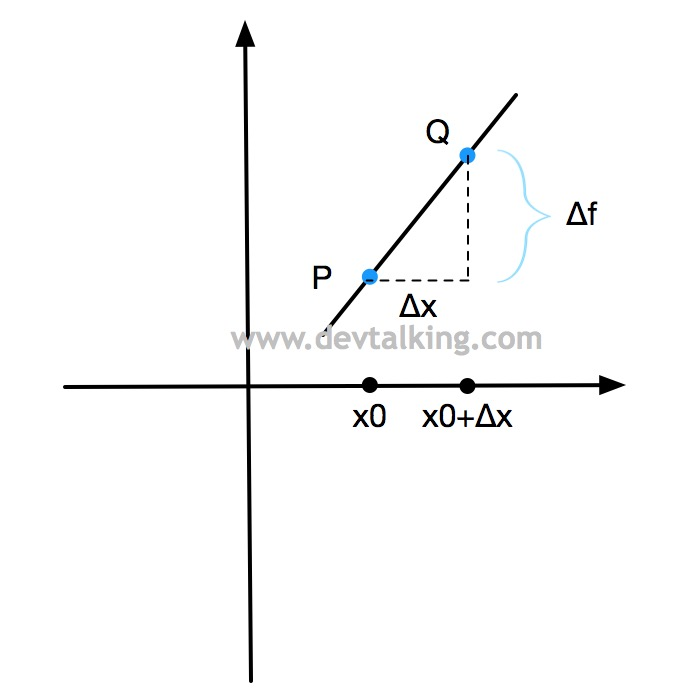$$k=\frac {f(x_0+\Delta x) - f(x_0)} {(x_0+\Delta x)-x_0}=\frac {f(x_0+\Delta x) - f(x_0)} {\Delta x}$$

$$k=\lim_{\Delta x->0}\frac {f(x_0+\Delta x) - f(x_0)} {\Delta x}$$

$$k=df=\lim_{\Delta x->0} \frac {f(x_0+\Delta x) - f(x_0)} {\Delta x}$$

极值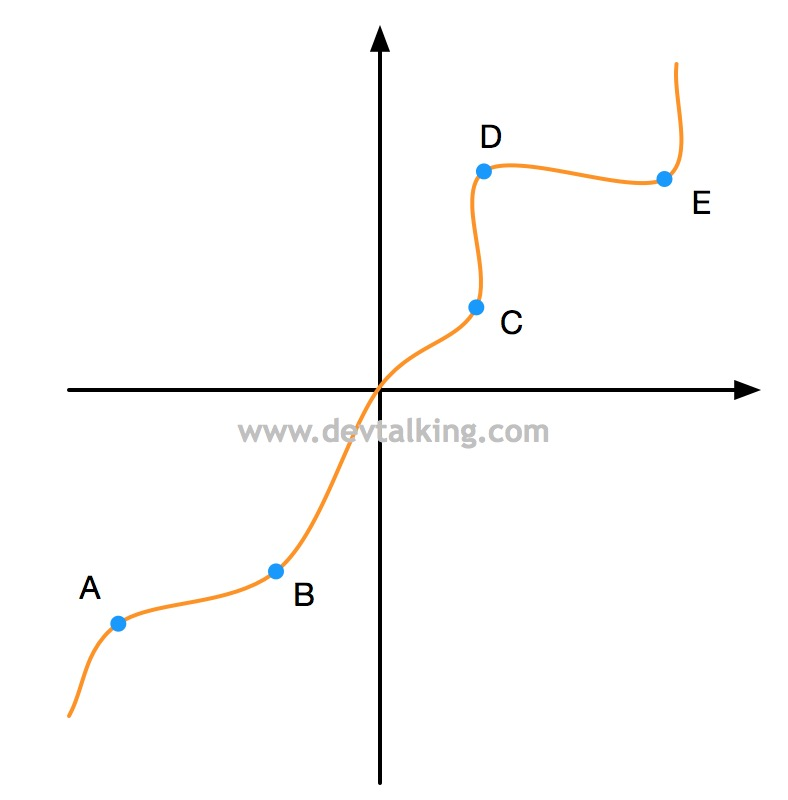$F(a,b)$的最小值

$$F(a,b) = \sum_{i=1}^n(y_i-(ax_i + b))^2 \\ =(y_1-(ax_1 + b))^2+(y_2-(ax_2 + b))^2+…+(y_n-(ax_n + b))^2 \\ =(y_1^2-2y_1(ax_1+b)+(ax_1+b)^2)+(y_2^2-2y_2(ax_2+b)+(ax_2+b)^2) \\ +…+(y_n^2-2y_n(ax_n+b)+(ax_n+b)^2) \\ =y_1^2-2ay_1x_1-2by_1+a^2x_1^2+2abx_1+b^2+y_2^2-2ay_2x_2-2by_2+a^2x_2^2+2abx_2+b^2 \\ +…+y_n^2-2ay_nx_n-2by_n+a^2x_n^2+2abx_n+b^2 \\ =(y_1^2+..+y_n^2)-2a(x_1y_1+..+x_ny_n)-2b(y_1+..+y_n) \\ +a^2(x_1^2+..+x_n^2)+2ab(x_1+..+x_n)+nb^2$$

$$\frac {(y_1^2+..+y_n^2)} n=\overline {y^2}$$

$$(y_1^2+..+y_n^2)=n\overline{y^2}$$

$$F(a,b)=n\overline{y^2}-2an\overline{xy}-2bn\overline y+a^2n\overline{x^2}+2abn\overline x+nb^2$$

$$\frac {\partial F}{\partial a}=-2n\overline xy+2an\overline {x^2}+2bn\overline x=0$$

$$\frac {\partial F}{\partial b}=-2n\overline y+2an\overline x+2nb=0$$

$$-\overline {xy}+a\overline {x^2}+b\overline x=0$$

$$-\overline y+a\overline x+b=0$$

$$a=\frac {\overline x \overline y-\overline {xy}} {(\overline x)^2-\overline {x^2}}$$

$$b=\overline y-a\overline x$$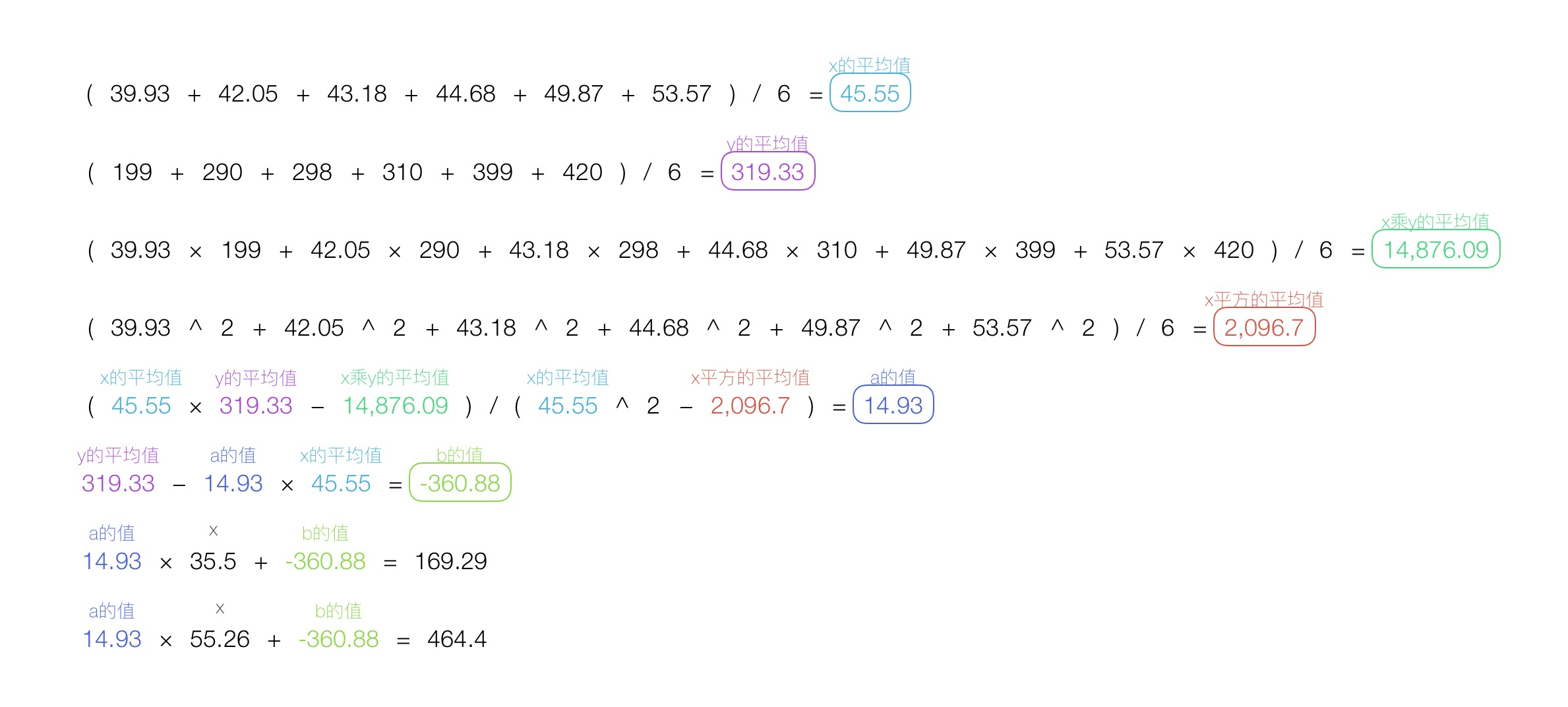评价回归线的拟合程度

总偏差平方和

$$SST=\sum_{i=1}^n(y_i-\overline y)^2$$

回归平方和

$$SSR=\sum_{i=1}^n(\hat y_i-\overline y)^2$$

误差平方和

$$SSE=SST-SSR$$